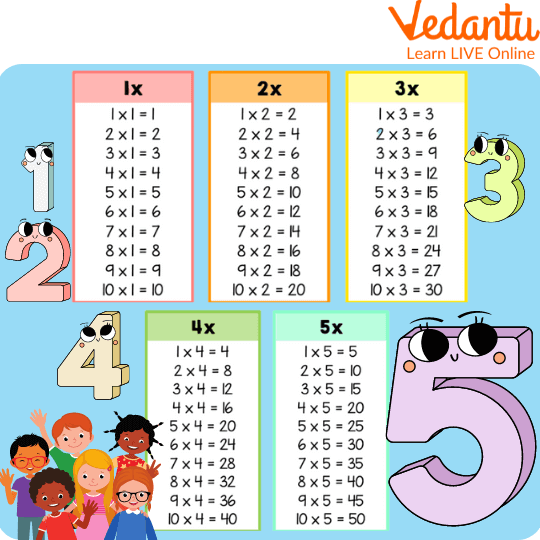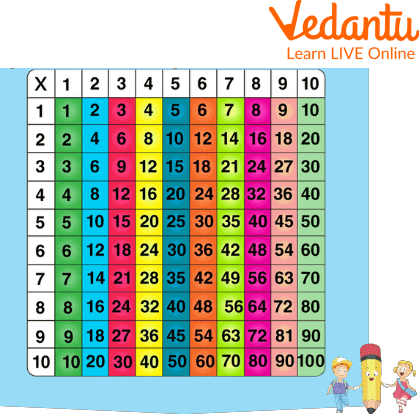Courses
Courses for Kids
Free study material
Offline Centres
More

# Multiplication Tables for KidsLast updated date: 27th Nov 2023
Total views: 100.2k
Views today: 1.00k## Spotlight on Multiplication Tables for Kids

In mathematics, multiplication is the repeated addition of a number. Before going into depth about the topic, Do you know what a multiplication table is? Why are they important? Let’s find out!

A multiplication table is a table which shows the product of two numbers(either two single-digit numbers or 1 single-digit number and one 2-digit number or two 2-digit numbers). It ranges from 1 1 = 1 up to 10 10 = 100 or even more because there is no limit to multiplication tables. In this article, we will learn 1 to 100 tables chart.

### Multiplication Tables for Kids

A multiplication table is a table which is used to define a multiplication operation for numbers which might be a 1-digit number, 2-digit number or even more. It is a list of products of a number.

One can obtain the multiplication table by multiplying a set of numbers with the other set of numbers.Multiplication Table

### Significance Of Multiplication Tables

Have you ever thought, What is so special about multiplication tables? Well, It is very time-consuming to add numbers over and over again. So, if we already know how many times 2 numbers can be multiplied together, we can easily find the product of those numbers! Isn’t it?

It will also help to do math faster and more accurately. Multiplication tables are like all math tables, which are drawn up using multiplication formulas. One of the most common formulas for multiplication is the equation “x” times “y”. Multiplication is also important in many everyday situations.

For kids, It could be helpful in calculations like dividing chocolates among siblings. In our daily life, there are many situations, such as cooking, gardening and collecting data about the number of people, that can be helpful from having a multiplication table. It can also help people calculate percentages and currency values.

### Multiplication Table

The multiplication tables of 1, 2, 5, and 10 are easier to remember as they follow a pattern.

• Any number multiplied by 1 will be equal to the number itself.

• If any number is multiplied by 2, it will be equal to double the number.

• The ones digits in the multiplication table of 5 alternate between 0 and 5.

• The table of 10 can be easily remembered because the digit at the one's place is always zero.

### 1 to 100 Tables Chart

With the help of a 1 to 100 tables chart, kids can easily understand multiplication tables.1 to 100 Tables Chart

### Classroom Activities

Learning multiplication tables becomes easier for children when they learn them in a fun way or the form of activities. Few classroom activities are there which can be performed in the classroom and will help kids to learn multiplication tables more easily.

Back to Back: This Game requires some extra brain power, as it requires finding a multiplier of the number instead of a product.

Materials Required: Blackboard or Whiteboard (with chalk or markers).

Steps

1. Ask two students to stand in front of the board facing away from each other.

1. At your “start “ signal, each student will write a number between 1-10 (you can increase the range) on the board.

1. Ask another student to come in front of the board, assume a number in mind, and announce the product of two numbers (make sure that the answer is correct).

1. If the first player can guess the other student’s assumed number, he wins the round.

1. The student who “won” the round will stay on the board, and the person who “loses” the round will be replaced with another student.

1. The student who won the most rounds in a row is the winner.

### Solved Examples

Find the product of the given numbers:

(a) $7 \times 7$

Ans. On multiplying 7 by 7,

It gives 49

(b) $12 \times 12$

Ans. On multiplying 12 by 12,

It gives 144

Therefore, the required answer is 144.

(c) $10 \times 11$

Ans. On multiplying 10 by 11,

It gives 110

Therefore, the required answer is 110.

### Practice Questions

Q 1. Find the product of the numbers given below:

(a) $10 \times 2$

(b) $12 \times 6$

(c) $15 \times 8$

Ans:

(a) 20

(b) 72

(c) 120

Q 2. Find the product of the numbers given below:

(a) $17 \times 9$

(b) $14 \times 6$

(c) $18 \times 8$

Ans:

(a) 153

(b) 84

(c) 144

Q 3. Find the product of the numbers given below:

(a) $20 \times 8$

(b) $44 \times 7$

(c) $98 \times 9$

Ans:

(a) 160

(b) 308

(c) 882

### Summary

In this article, we have learned about multiplication tables, their definition, and their significance. We also learned that it is much easier to learn tables 1,2,5, and 10 because any number multiplied by 1 gives the number itself as an answer. We also learned about the importance of multiplication tables; what is the impact of multiplications in our lives? We also learnt some classroom activities which will help to learn multiplication tables very easily. With the help of 1 to 100 table charts, we learned multiplication. We have also done some questions to understand the concept very well and practised some questions, which will help us grasp the concept more easily.

## FAQs on Multiplication Tables for Kids

1. How many years ago the first multiplication chart was created?

The first multiplication chart was created 4000 years ago approx.

2. Write the parts of multiplication.

The parts of a multiplication sentence are the multiplicand, multiplier, and product. The multiplicand is the first number, the multiplier is the second number, and the product is the answer.

3. Multiplication Problems can be named as _____?

Multiplication Problems can be named Products.